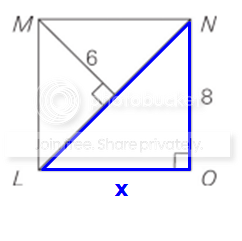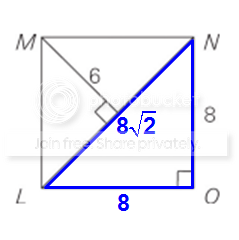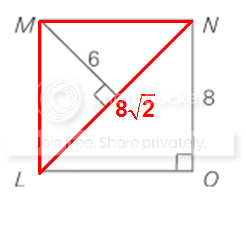GMAT Question of the Day: Daily via email | Daily via Instagram New to GMAT Club? Watch this Video

 It is currently 08 Apr 2020, 15:04### GMAT Club Daily Prep

#### Thank you for using the timer - this advanced tool can estimate your performance and suggest more practice questions. We have subscribed you to Daily Prep Questions via email.

Customized
for You

we will pick new questions that match your level based on your Timer History

Track

every week, we’ll send you an estimated GMAT score based on your performance

Practice
Pays

we will pick new questions that match your level based on your Timer History

#### Not interested in getting valuable practice questions and articles delivered to your email? No problem, unsubscribe here.# In the diagram above, if the area of triangle LNO is 32, then what is

Author Message
TAGS:

### Hide Tags

Math ExpertV
Joined: 02 Sep 2009
Posts: 62637
In the diagram above, if the area of triangle LNO is 32, then what is  [#permalink]

### Show Tags

1
400:00

Difficulty:45% (medium)

Question Stats:66% (01:55) correct34% (01:57) wrongbased on 214 sessions

### HideShow timer StatisticsIn the diagram above, if the area of triangle LNO is 32, then what is the area of triangle LMN?

A. 24
B. $$24 \sqrt{2}$$
C. $$24 \sqrt{3}$$
D. 32
E. 48

Attachment:2016-01-04_0017.png [ 10.43 KiB | Viewed 3281 times ]

_________________
ManagerJoined: 09 Jul 2013
Posts: 106
Re: In the diagram above, if the area of triangle LNO is 32, then what is  [#permalink]

### Show Tags

2
Bunuel wrote:In the diagram above, if the area of triangle LNO is 32, then what is the area of triangle LMN?

A. 24
B. $$24 \sqrt{2}$$
C. $$24 \sqrt{3}$$
D. 32
E. 48

Even though the figure looks like a square, that can NEVER be assumed. It explicitly states that the figure is not drawn to scale (something we should assume all the time unless it is stated otherwise), and we must ONLY work with the information given.

Examining triangle LNO, we are given that the

$$area= \frac{base * height}{2} = 32$$

Since we have a right angle at O, we can consider the base to be LO and the height to be ON. We are given the height = 8, so the base, LO, is $$\frac{area*2}{base} = \frac{32*2}{8}=8$$

So, triangle LNO is an isosceles right triangle, and therefore the hypotenuse, LN, is $$8\sqrt{2}$$

Now looking at triangle LMN, we have the height given as 6, and the base LN calculated as $$8\sqrt{2}$$, so

$$area=\frac{6*8\sqrt{2}}{2} = 24\sqrt{2}$$

_________________
Dave de Koos
GMAT Club LegendV
Joined: 11 Sep 2015
Posts: 4604
GMAT 1: 770 Q49 V46
Re: In the diagram above, if the area of triangle LNO is 32, then what is  [#permalink]

### Show Tags

Top Contributor
Bunuel wrote:In the diagram above, if the area of triangle LNO is 32, then what is the area of triangle LMN?

A. 24
B. $$24 \sqrt{2}$$
C. $$24 \sqrt{3}$$
D. 32
E. 48

Attachment:
2016-01-04_0017.png

First examine the blue triangle.We're told the area = 32
Area = (base)(height)/2
So, 32 = (x)(8)/2
Solve to get: x = 8

Since the blue triangle is a right triangle, we can apply the Pythagorean Theorem to find the length of the hypotenuse.
We get: 8² + 8² = hypotenuse²
Simplify: 128 = hypotenuse²
So, hypotenuse = √128 = 8√2

We get:At this point, we can focus on the red triangleWe can see that we now have the length of the base AND the height.
So, area of red triangle = (base)(height)/2
= (8√2)(6)/2
= 24√2

RELATED VIDEOS

_________________
InternB
Joined: 23 Feb 2019
Posts: 1
Re: In the diagram above, if the area of triangle LNO is 32, then what is  [#permalink]

### Show Tags

Shouldn't the answer be 32 as the two triangles must be congruent and hence have the same area?
ManagerJoined: 09 Jul 2013
Posts: 106
Re: In the diagram above, if the area of triangle LNO is 32, then what is  [#permalink]

### Show Tags

RanjaniRaghupathi wrote:
Shouldn't the answer be 32 as the two triangles must be congruent and hence have the same area?

Why do you think the two triangles are congruent? We were never told that LMNO is a rectangle. This is a great example of "Note: figure not drawn to scale" being very important.

Remember, no assumptions. Use only the information provided and what can be inferred from it.

Posted from my mobile device
_________________
Dave de KoosRe: In the diagram above, if the area of triangle LNO is 32, then what is   [#permalink] 24 Feb 2019, 10:53
Display posts from previous: Sort by

# In the diagram above, if the area of triangle LNO is 32, then what is